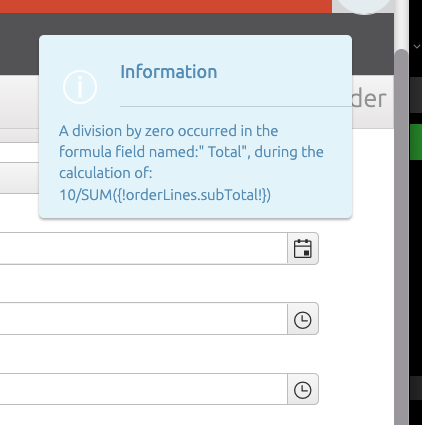# Formula field

The Formula field gives the possibility to calculate field values with formulas.

### Properties

Setting Required Description
Label Yes The label is used for users of BizzStream. The label is shown in documents left to the value of the field. Labels can always be edited.
Translation key No Can be used to replace the field label with a translation
Name Yes The name is a BizzStream systemfield. Once the Document Definition is persisted, this field cannot be edited anymore.
Decimal Yes Set the number of decimal values. The value can be 0 to infinite.
Formula Yes Insert the formula you want to be calculated. This field can contain BizzStream Expressions.

### How the data is stored

Text fields will be saved accordingly in the database:

``````formulaField: 9.891
``````

### Formulas in fields

Operator Description
- subtraction
* multiplication
/ division
`{!source.fieldName!}` reference to a field in the same document.
SUM() Sum of all values
MAX() The maximum value
AVG() The average value
MIN() The minimum value

You can add simple mathematic formulas. For example:

``````6 * 20 + 12/3 - 1
``````

In combination with BizzStream Expressions the formula field can be used to calculate values of referenced fields.

``````6 * (1 - {!source.discount!} / 100)
``````

In which {!source.discount!} is a reference to a the field discount.

You can use multiple expressions at once, for example:

``````{!source.subTotal!} * (1 - {!source.discount!} / 100)
``````

In which the subtotal is multiplied with the discount percentage to find the total discount amount.

It is also possible to use the field value of a referenced document, for example:

``````{!source.article{"fieldName":"price"}!} * (1 - {!source.discount!}/100)
``````

The document article contains a field with the name price.

In the case of lines, you can sum fields with the SUM operator. For example:

``````SUM({!invoiceLine.amount!})
``````

this formula adds all amount fields in the invoiceLine. (MIN, MAX en AVG can be used in a similar fashion to calculate respectively the minimum, maximum and average value)

### Formulas in rules

You can use formulas in rules as well. If you want to refer to a field in the same line use the prefix sourceLine:

``````{!sourceLine.price!} * (1 - {!source.discount!} / 100)
``````

With this formula the price of a rule is multiplied by the discount percentage of the headerfield discount.

### Formulas and time fields

The value of a time field can be used in formulas. The value of a time field has to be converted to a minute or hour value. For example the value of 12:03 PM is 723 minutes or 12,05 hours. Converting the value of a time field can be achieved by:

``````{!fieldName{"format":"hours"}!}
``````

In this example you can replace hours with minutes. With this numeric value the formula field can calculate.

For example, to calculate the time between start and finish:

``````{!finish{"format":"minutes"}!} - {!start{"format":"minutes"}!}
``````

### Division by 0 in formula fields

Formula fields can depend on other field(s) value. When the value(s) on which a formula depends become 0 this can cause the computation of the formula expression to contain a division by 0. In such cases the formula field will remain empty in the user interface, however such cases are detected and a message with more detailed information about the field and the expression in which this happened is dysplayed in the browser console.This information can be useful to understand and, if necessary, correct document data.